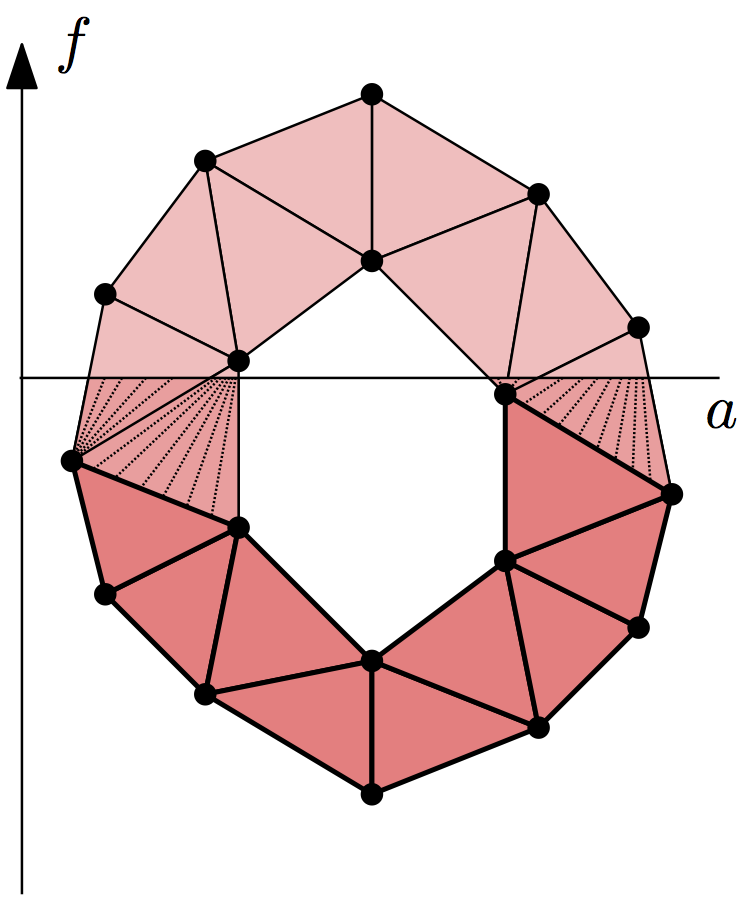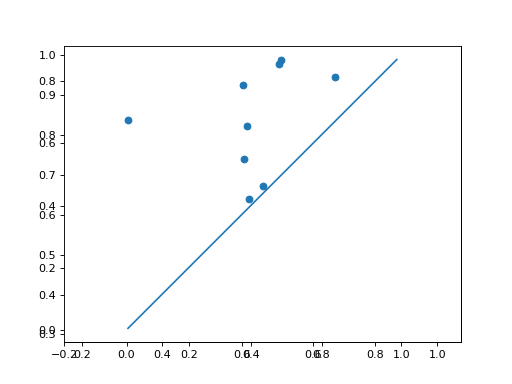# Lower-star Filtrations¶

Given a simplicial complex $$K$$ and a real-valued function defined on its vertices, $$f: \operatorname{Vrt} K \to \mathbb{R}$$, we can extend it to a continuous function by linear interpolation on the interiors of the simplices. Suppose we want to compute persistent homology of the sublevel sets of this function, $$|K|_a = f^{-1}(-\infty, a]$$. Its persistence diagrams are the same as for the lower-star filtration of simplicial complexes, $$K_a = \{ \sigma \in K \mid \max_{v \in \sigma} f(v) \leq a \}$$. (It’s easy to verify that the inclusion $$K_a \subseteq |K|_a$$ is a homotopy equivalence.)So given such a function $$f$$, it suffices to assign to each simplex of $$K$$ the value of its maximum vertex. Sorting the resulting filtration creates the lower-star filtration. To construct the symmetric upper-star filtration, assign the minimum of the vertices as the simplex data and pass reverse = True to sort().

Dionysus provides a helper function, fill_freudenthal(), to build lower-star and upper-star filtrations of the Freudenthal triangulation on a grid, with values given in a NumPy array.

Take a random NumPy array:

>>> a = np.random.random((10,10))
>>> plt.matshow(a)
<...>
>>> plt.colorbar()
<...>


(png, hires.png, pdf)Use fill_freudenthal() to construct the triangulation:

>>> f_lower_star = d.fill_freudenthal(a)
>>> f_upper_star = d.fill_freudenthal(a, reverse = True)


Compute persistence as usual:

>>> p = d.homology_persistence(f_lower_star)
>>> dgms = d.init_diagrams(p, f_lower_star)


Use Plotting functionality to plot the diagrams:

>>> d.plot.plot_diagram(dgms)
>>> d.plot.plot_diagram(dgms)


(png, hires.png, pdf)